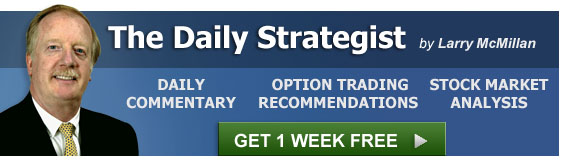Volatility Skew Information | Option Strategist
Home » Blog » 2013 » 03 » Volatility Skew Information##### By Lawrence G. McMillan

The term "volatility skew" refers to the situation where individual options on a particular entity have different implied volatilities that form a pattern. The pattern usually takes one of two forms: either the higher strikes have the higher implied volatilities (a forward or positive skew) or the lower strikes have the higher implied volatilities (a reverse or negative skew). Most of the time, \$OEX, \$SPX and other index options have a negative skew – that is out-of-the-money puts are much more expensive than out-of-the-money calls. The most common place to find a positive skew is in the futures markets, particularly the grains (Corn, Wheat, or Soybeans) although others such as Coffee, Sugar, and so on normally have a positive skew as well.

There is also a horizontal skew: that is, longer-term options generally trade with lower implied volatilities than do short-term options. This particular type of skew is just a fact of life, reflecting the difficulty of making longer-term volatility projections.

The theory behind "trading the skew" is that you are getting a theoretical advantage by essentially buying and selling options on the same entity (the underlying), yet these options have different volatility projections for that single underlying. Obviously, those volatilities must eventually converge – one option is, by definition, overpriced with respect to the other. There are some subtleties to this theory, but the general idea is a valid one.

For those wanting more background and education on volatility skews and how to trade them, I suggest you consider taking our online option seminar on the subject, "Volatility Trading Part 2."

For those looking for lists of stocks and futures with skewed options, we publish such data daily on The Strategy Zone.  The following is a small excerpt of how the data looks:

 Stock Price TotOptVolu Put Volu Implied VolofIV Skew Stk Hi Stk Lo Stk Last \$OEX: 576.680 22933 14034 16.5325 4.8467 29.32 577.87 570.46 576.68 \$SOX: 609.970 7050 5325 46.2085 3.9177 8.48 614.13 581.73 609.97

Reading from left to right, the symbol is shown (index symbols begin with \$, and futures symbols with @), followed by the closing price, the total option volume and the put volume traded. Then the composite implied volatility is shown. The next two numbers are the important ones as far as identifying the volatility skew, if it exists. Actually, everything in this list has some sort of a skew, so you can assume that it exists if it's in this report.

First there is a number called Volatility of Implied Volatility (VolofIV). This is nothing more than the standard deviation of the implied volatilities on this entity. The number next to it (Skew) normalizes the number, by dividing it by the composite implied volatility. The larger these numbers, the more skewed the options are. The second number (Skew) is probability the more important one, since this number can be compared with other stocks, indices, and futures. It is really the Volatility of the Implied Volatility for all the individual options on this entity.

#### Using the List

You will normally see that the list is dominated by futures and index options – as they have the most common skews. Occasionally some stock options will creep into the top of the list as well.  One must first ask questions, such as "How is the skew distributed?" Is it horizontal? That is, are the near-term options trading with a much higher implieds, and thus distorting things? If so, calendar spreads might be your preferred strategy. On the other hand, if the skew is vertical, you will notice the implieds either uniformly getting larger or smaller as you look at the strikes, reading from lowest to highest.  Assuming you find a skew you like, the next paragraph presents the general rules for trading it.

#### Assuming the skew is vertical, then:

• If the skew is positive and the composite implied volatility is in a low percentile (if you don't understand all these terms, see our Volatility Data Guide), consider Put backspreads as a strategy
• If the skew is negative and the composite implied volatility is in a low percentile, consider Call backspreads as a strategy.
• If the skew is positive and the composite implied volatility is in a very high percentile, then consider Call ratio spreads as a strategy.
• If the skew is negative and the composite implied volatility is in a very high percentile, then consider Put ratio spreads as a strategy.

Call and put ratio spreads involve naked options, and thus have theoretically large or even unlimited risk. As such, they are not suitable for all traders.  Be sure you know what you're doing before getting into those strategies.

Backspreads, on the other hand, have limited risk – although you could lose 100% of the margin you put forth. A typical backspread example, with XYZ stock at 100, might be to buy 2 XYZ July 100 calls and sell 1 XYZ July 90 call.

Again, if you're at all uncertain about this concept (trading the volatility skew) or its implementation via the above strategies, do more reading or take the course on the subject. The strategy is discussed at length in both of the books, Options As A Strategic Investment and McMillan On Options

For volatility skew trading candidates on a daily basis, subscribe to The Daily Strategist.